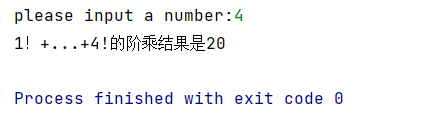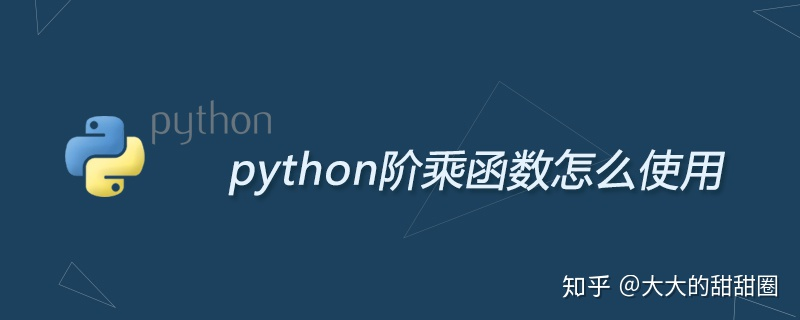• 在本篇文章里小编给大家整理的是关于Python阶乘求和的代码实例，有需要的朋友们可以跟着学习下。
• 今天小编就为大家分享一篇python 阶乘累加和的实例，具有很好的参考价值，希望对大家有所帮助。一起跟随小编过来看看吧
• Python阶乘求和的方法 题目描述： 获得用户输入的整数n，输出 1!+2!+…+n!的值。 如果输入数值为0、负数、非数字或非整数，输出提示信息：输入有误，请输入正整数。 （推荐学习：Python视频教程） 方法一： #...Python阶乘求和的方法
题目描述：
获得用户输入的整数n，输出 1!+2!+…+n!的值。
如果输入数值为0、负数、非数字或非整数，输出提示信息：输入有误，请输入正整数。
（推荐学习：Python视频教程）
方法一：
#factTest1
def main():
a = input()
sum = 0
if a.isdigit():
n = eval(a)
if n > 0:
fact = 1
for i in range(1, n+1):
fact *= i
sum += fact
print(sum)
else:
print("输入有误，请输入正整数")
else:
print("输入有误，请输入正整数")
main()

方法二：递归思想
#factTest2
import sys
sys.setrecursionlimit(5000)
def getSum(i):
sum = 0
if i==0:
return 0
else:
for x in range(1,i+1):
sum += fact(x)
return sum
def fact(m):
if m==0:
return 1
else:
return m*fact(m-1)
def main():
n = input()
if n.isdigit():
a = eval(n)
if a>0:
result = getSum(a)
print(result)
else:
print("输入有误，请输入正整数")
else:
print("输入有误，请输入正整数")
main()

问题总结：
当使用递归方法求1024的阶乘时，出现了一个异常：RecursionError: maximum recursion depth exceeded in comparison，超出了递归的最大深度。一些网友提到Python中默认的最大递归深度为1000，但在实际测试中，我的电脑到997的时候就出现异常了，不知道这是由什么决定的。因此，为了能够计算1024的阶乘，需要为最大递归深度赋予一个更大的值。这里可以采用以下方法：
import sys
sys.setrecursionlimit(5000) #修改为5000

另外，也可以查看最大递归深度：
import sys
sys.getrecursionlimit()  # output:1000

相关免费资料分享（点击即可免费观看~）
1、数据分析_地震–Python实战之地震预测
http://www.makeru.com.cn/live/5020_2148.html?s=96806
2、数据分析_选股–Python项目实战：智能选股（股票）
http://www.makeru.com.cn/live/5020_2158.html?s=96806
3、旅游数据分析–掌握Python工具，全国上榜名吃尽在手中
http://www.makeru.com.cn/live/5020_2154.html?s=96806
142244252 学习资料交流群，想要和志同道合的朋友一起学习，大家互相分享自己的学习资料和作品，欢迎感兴趣的朋友共同学习，共同进步，每天还会有免费的公开课程！！
展开全文机器学习 人工智能 大数据 深度学习
• 依次计算每个阶乘的值，然后相加起来，即可得到结果 代码： # 获得用户输入的整数n，输出 1!+2!+…+n!的值。 num = int(input("please input a number:")) num_sum = 0 for i in range(1,num+1): for j in range(1...
题目： 获得用户输入的整数n，输出 1!+2!+…+n!的值。
思路： 依次计算每个阶乘的值，然后相加起来，即可得到结果
代码：
# 获得用户输入的整数n，输出 1!+2!+…+n!的值。
num = int(input("please input a number:"))

num_sum = 0
for i in range(1,num+1):
for j in range(1,i+1):
num_sum = num_sum+ j
print(f"1！+...+{num}!的阶乘结果是{num_sum}")


运行截图：展开全文• 输入一个正整数num，计算这个正整数的阶乘，并将计算结果输出。 # 请使用 input() 输入一个正整数 num num=int(input()) # 请计算这个正整数的阶乘，并将计算结果输出 count=1 for i in range(num,1,-1): count=...
请用程序实现 输入一个正整数num，计算这个正整数的阶乘，并将计算结果输出。
# 请使用 input() 输入一个正整数 num
num=int(input())
# 请计算这个正整数的阶乘，并将计算结果输出
count=1
for i in range(num,1,-1):
count=count*i
print(count)
展开全文• python中两种阶乘函数有两种阶乘函数，自己创建的不算。 一是numpy库中的阶乘函数 1 2 improt numpyprint numpy.math.factorial(3) ...

python中两种阶乘函数有两种阶乘函数，自己创建的不算。一是numpy库中的阶乘函数
1
2
improt numpyprint numpy.math.factorial(3)
二是python 自带的标准库也有阶乘函数
1
2
import mathprint math.factorial(3)
例：
1
2
3
4
#由于第一种需要下载库，我们使用第二种 import math value = math.factorial(3)  print(value)
输出：
1
6

以上就是python阶乘函数怎么使用的详细内容
如果大家如果在学习中遇到困难，想找一个Python学习交流环境，可以加入我们的Python学习圈，点击我加入吧，会节约很多时间，减少很多遇到的难题。

展开全文• def factorial(n): if n==0: return 1 else: recurse=factorial(n-1) result=n*recurse return result def fact(n): s=1 for i in range(1,n+1): s*=...
• print(math.factorial(3)) #3*2=6
• 第三种直接定义一个阶乘函数，随时都可以调用，从而得到不同值。方法一：普通方法：a = 1n = 5for i in range(1,n+1):a = a * iprint(a)20方法二：reduce()函数：from functools import reducen = 5print(...
• 在本篇文章里小编给各位整理的是关于python计算n的阶乘的相关知识点，需要的朋友们参考下。
• 整数的阶乘（英语：factorial）是所有小于及等于该数的正整数的积，0的阶乘为1。即：n!=1×2×3×...×n。 # 通过用户输入数字计算阶乘 # 获取用户输入的数字 num = int(input("请输入一个数字: ")) factorial =...软件测试 经验分享
• python阶乘末尾0的个数python写一个脚本，使得可以实现下列功能： 输入一个正整数，这个数在1至1000； 输出这个数的阶乘； 输出这个数的阶乘末尾有几个0； 这个脚本如下：def multi(x, y): return x * y def Zero...
• #Python3 实例--Python 阶乘实例： print("Python3 实例--Python 阶乘实例：") #原则：整数的阶乘（英语：factorial）是所有小于及等于该数的正整数的积，0的阶乘为1。即：n!=1×2×3×...×n def jieceng(x): ...
• 1、递归的特点 递归算法是一种直接或间接调用自身算法的过程，在计算机编程中，递归算法对解决一大类问题是十分，它往往使算法的描述简洁而且易于理解。 递归算法解决问题的特点： （1）递归就是在过程或函数里...
• 阶乘 def jiecheng(n) : if n == 0 : return 1 else : return n * jiecheng(n-1) print(jiecheng(20))
• # python 阶乘计算器 i = 1 result = 1 while i <= 20: print(i) result = result * i print(result) i = i+1 运行结果： 1 1 2 2 3 6 4 24 5 120 6 720 7 5040 8 40320 9 362880 10 3628800 11 39916800.....练习
• ## python阶乘和

千次阅读 2018-07-26 12:01:19
def jiechenghe(n): if n == 1: return 1 elif n == 2: return 3 return jiechenghe(n - 1) + n * (jiechenghe(n - 1) - jiechenghe(n - 2)) print(jiechenghe(2))
• 主要介绍了详解用python计算阶乘的几种方法，文中通过示例代码介绍的非常详细，对大家的学习或者工作具有一定的参考学习价值，需要的朋友们下面随着小编来一起学习学习吧
• ''' ...阶乘 ''' n = int(input("请输入一个正整数:")) ji = 1 for i in range(1,n+1): ji *= i print(ji) 定义函数方法 def jiecheng(n): ji = 1 for x in range(1,n+1): ji *= x return...
• python阶乘怎么表示I am currently working on an article about calculating sines and cosines using Taylor Polynomials. These make heavy use of factorials so I started thinking about ways to streamline...
• 基础训练BASIC-30 阶乘计算 问题描述 　输入一个正整数n，输出n!的值。 　其中n!=123*…*n。 算法描述 　n!可能很大，而计算机能表示的整数范围有限，需要使用高精度计算的方法。使用一个数组A来表示一个大整数a，A...
• ## Python的阶乘求和

千次阅读 2021-02-05 17:04:33
题目描述： 获得用户输入的整数n，输出 1!+2!+…+n!的值。 如果输入数值为0、负数、非数字或非...一些网友提到Python中默认的最大递归深度为1000，但在实际测试中，到997的时候就出现异常了，不知道这是由什么决定的数据分析 数据挖掘 线性代数 爬虫
• ## python的阶乘

千次阅读 2019-08-03 10:26:00递归 算法...

# python阶乘python 订阅